# 10.3 Parameters and Parameter Elimination

$$\newcommand{\vecs}{\overset { \rightharpoonup} {\mathbf{#1}} }$$ $$\newcommand{\vecd}{\overset{-\!-\!\rightharpoonup}{\vphantom{a}\smash {#1}}}$$$$\newcommand{\id}{\mathrm{id}}$$ $$\newcommand{\Span}{\mathrm{span}}$$ $$\newcommand{\kernel}{\mathrm{null}\,}$$ $$\newcommand{\range}{\mathrm{range}\,}$$ $$\newcommand{\RealPart}{\mathrm{Re}}$$ $$\newcommand{\ImaginaryPart}{\mathrm{Im}}$$ $$\newcommand{\Argument}{\mathrm{Arg}}$$ $$\newcommand{\norm}{\| #1 \|}$$ $$\newcommand{\inner}{\langle #1, #2 \rangle}$$ $$\newcommand{\Span}{\mathrm{span}}$$ $$\newcommand{\id}{\mathrm{id}}$$ $$\newcommand{\Span}{\mathrm{span}}$$ $$\newcommand{\kernel}{\mathrm{null}\,}$$ $$\newcommand{\range}{\mathrm{range}\,}$$ $$\newcommand{\RealPart}{\mathrm{Re}}$$ $$\newcommand{\ImaginaryPart}{\mathrm{Im}}$$ $$\newcommand{\Argument}{\mathrm{Arg}}$$ $$\newcommand{\norm}{\| #1 \|}$$ $$\newcommand{\inner}{\langle #1, #2 \rangle}$$ $$\newcommand{\Span}{\mathrm{span}}$$$$\newcommand{\AA}{\unicode[.8,0]{x212B}}$$

In a parametric equation, the variables $$x$$ and $$y$$ are not dependent on one another. Instead, both variables are dependent on a third variable, $$t$$. This is the parameter or a number that affects the behavior of the equation. Usually $$t$$ will stand for time. A real world example of the relationship between $$x, y$$ and $$t$$ is the height, weight and age of a baby.

Both the height and the weight of a baby depend on time, but there is also clearly a positive relationship between just the height and weight of the baby. By focusing on the relationship between the height and the weight and letting time hide in the background, you create a parametric relationship between the three variables.

What other types of real world situations are modeled with parametric equations?

## Eliminating the Parameter

In your graphing calculator there is a parametric mode. Once you put your calculator into parametric mode, on the graphing screen you will no longer see $$y=$$ ___, instead, you will see:

##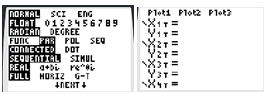Notice how for plot one, the calculator is asking for two equations based on variable $$T$$ :

$$x_{1 T}=f(t)$$

$$y_{1 T}=g(t)$$

This is called parametric form. Parametric form refers to a relationship that includes $$x=f(t)$$ and $$y=g(t)$$. In order to transform a parametric equation into a normal one, you need to do a process called "eliminating the parameter." "Eliminating the parameter" is a phrase that means to turn a parametric equation that has $$x=f(t)$$ and $$y=g(t)$$ into just a relationship between $$y$$ and $$x$$. You are eliminating $$t$$. To do this, you must solve the $$x=f(t)$$ equation for $$t=f^{-1}(x)$$ and substitute this value of $$t$$ into the $$y$$ equation. This will produce a normal function of $$y$$ based on $$x$$.There are two major benefits of graphing in parametric form. First, it is straightforward to graph a portion of a regular function using the $$T_{\min }, T_{\max }$$ and $$T_{\text {step }}$$ in the window setting. Second, parametric form enables you to graph projectiles in motion and see the effects of time.

A tortoise and a hare start 202 feet apart and then race to a flag halfway between them. The hare decides to take a nap and give the tortoise a 21 second head start. The hare runs at 9.8 feet per second and the tortoise hustles along at 3.2 feet per second. This situation can be represented by parametric equations and we can use the equations to determine who wins this epic race and by how much.

First draw a picture and then represent each character with a set of parametric equations.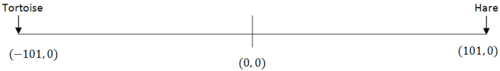The tortoise's position is (-101,0) at $$t=0$$ and (-97.8,0) at $$t=1$$. You can deduce that the equation modeling the tortoise's position is:

$$x_{1}=-101+3.2 \cdot t$$

$$y_{1}=0$$

The hare's position is (101,0) at $$t=21$$ and (91.2,0) at $$t=22$$. Note that it does not make sense to make equations modeling the hare's position before 21 seconds have elapsed because the Hare is napping and not moving. You can set up an equation to solve for the hare's theoretical starting position had he been running the whole time.

$$x_{2}=b-9.8 t$$

$$101=b-9.8 \cdot 21$$

$$305.8=b$$

The hare's position equation after $$t=21$$ can be modeled by:

$$x_{2}=305.8-9.8 \cdot t$$

$$y_{2}=0$$

The tortoise crosses $$x=0$$ when $$t \approx 31.5$$. The hare crosses $$x=0$$ when $$t \approx 31.2$$. The hare wins by about 1.15 feet.

Now, use your calculator to display these parametric equations. $$T$$

here are many settings you should know for parametric equations that bring questions like this to life. The TI-84 has features that allow you to see the race happen.

First, set the mode to simultaneous graphing. This will show both the tortoise and hare's position at the same time.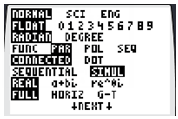Next, change the graphing window so that $$t$$ varies between 0 and 32 seconds. The $$T_{\text {step }}$$ determines how often the calculator will calculate points. The larger the $$T_{\text {step }}$$, the faster and less accurately the graph will plot. Also change the $$x$$ to vary between -110 and 110 so you can see the positions of both characters.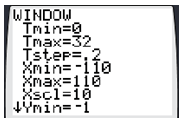Input the parametric equations. Toggle to the left of the $$x$$ and change the cursor from a line to a line with a bubble at the end. This shows their position more clearly.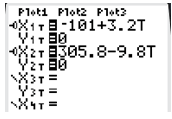Now when you graph you should watch the race unfold as the two position graphs race towards each other.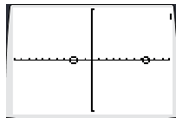## Examples

##### Example 1

Earlier, you were asked what types of real world situations can be modeled by parametric equations. Parametric equations are often used when only a portion of a graph is useful. By limiting the domain of $$t$$, you can graph the precise interval of the function you want. Parametric equations are also useful when two different variables jointly depend on a third variable and you wish to look at the relationship between the two dependent variables. This is very common in statistics where an underlying variable may actually be the cause of a problem and the observer can only examine the relationship between the outcomes that they see. In the physical world, parametric equations are exceptional at graphing position over time because the horizontal and vertical vectors of objects in free motion are each dependent on time, yet independent of one another.

##### Example 2

Eliminate the parameter in the following equations.

$$x=6 t-2$$

$$y=5 t^{2}-6 t$$

$$x=6 t-2$$ So $$\frac{x+2}{6}=t .$$ Now, substitute this value for $$t$$ into the second equation:

$$y=5\left(\frac{x+2}{6}\right)^{2}-6\left(\frac{x+2}{6}\right)$$

##### Example 3

For the given parametric equation, graph over each interval of $$t$$.

$$x=t^{2}-4$$

$$y=2 t$$

1. $$-2 \leq t \leq 0$$

2. $$0 \leq t \leq 5$$

3. $$-3 \leq t \leq 2$$

a. A good place to start is to find the coordinates where $$t$$ indicates the graph will start and end. For $$-2 \leq t \leq 0, t=-2$$ and $$t=0$$ indicate that the points (0,-4) and (-4,0) are the endpoints of the graph.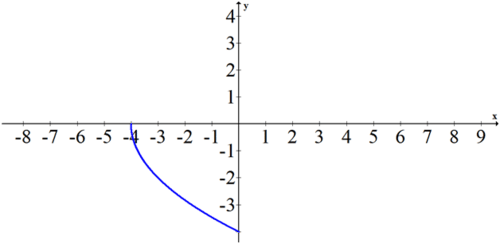b. $$0 \leq t \leq 5$$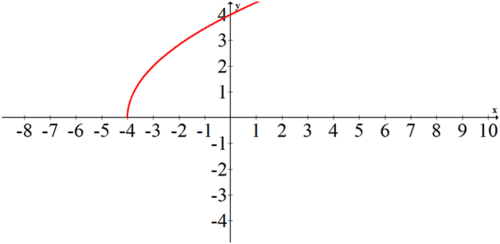c. $$-3 \leq t \leq 2$$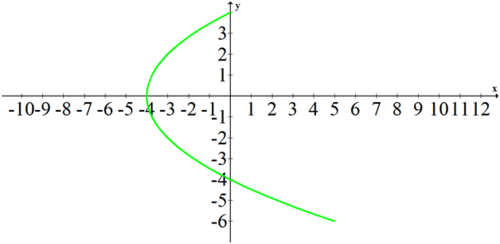##### Example 4

Eliminate the parameter and graph the following parametric curve.

$$x=3 \cdot \sin t$$

$$y=3 \cdot \cos t$$

When parametric equations involve trigonometric functions you can use the Pythagorean Identity, $$\sin ^{2} t+\cos ^{2} t=1 \cdot$$ In this problem, $$\sin t=\frac{x}{3}$$ (from the first equation) and $$\cos t=\frac{y}{3}$$ (from the second equation). Substitute these values into the Pythagorean Identity and you have:

$$\left(\frac{x}{3}\right)^{2}+\left(\frac{y}{3}\right)^{2}=1$$

$$x^{2}+y^{2}=9$$

This is a circle centered at the origin with radius $$3 .$$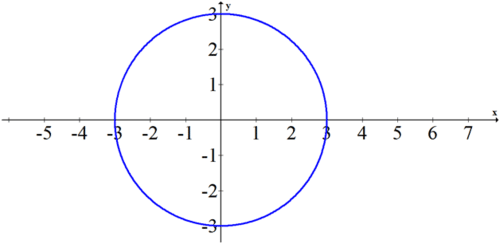##### Example 5

Find the parameterization for the line segment connecting the points (1,3) and $$(4,8) .$$

Use the fact that a point plus a vector yields another point. A vector between these points is $$<4-1,8-3>=<3,5>$$

Thus the point (1,3) plus $$t$$ times the vector $$<3,5>$$ will produce the point (4,8) when $$t=1$$ and the point (1,3) when $$t=0$$.

$$(x, y)=(1,3)+t \cdot<3,5>,$$ for $$0 \leq t \leq 1$$

You than then break up this vector equation into parametric form.

$$x=1+3 t$$

$$y=3+5 t$$

$$0 \leq t \leq 1$$

##### Review

Eliminate the parameter in the following sets of parametric equations.

1. $$x=3 t-1 ; y=4 t^{2}-2 t$$

2. $$x=3 t^{2}+6 t ; y=2 t-1$$

3. $$x=t+2 ; y=t^{2}+4 t+4$$

4. $$x=t-5 ; y=t^{3}+1$$

5. $$x=t+4 ; y=t^{2}-5$$

For the parametric equation $$x=t, y=t^{2}+1,$$ graph over each interval of $$t$$.

6. $$-2 \leq t \leq-1$$

7. $$-1 \leq t \leq 0$$

8. $$-1 \leq t \leq 1$$

9. $$-2 \leq t \leq 2$$

10. $$-5 \leq t \leq 5$$

11. Eliminate the parameter and graph the following parametric curve:

$$x=\sin t, y=-4+3 \cos t$$

12. Eliminate the parameter and graph the following parametric curve:

$$x=1+2 \cos t, y=1+2 \sin t$$

13. Using the previous problem as a model, find a parameterization for the circle with center (2, 4) and radius $$3 .$$

14. Find the parameterization for the line segment connecting the points (2,7) and $$(1,4) .$$

15. Find a parameterization for the ellipse $$\frac{x^{2}}{4}+\frac{y^{2}}{25}=1$$. Use the fact that $$\cos ^{2} t+\sin ^{2} t=1$$. Check your answer with your calculator.

16. Find a parameterization for the ellipse $$\frac{(x-4)^{2}}{9}+\frac{(y+1)^{2}}{36}=1$$. Check your answer with your calculator.

This page titled 10.3 Parameters and Parameter Elimination is shared under a CK-12 license and was authored, remixed, and/or curated by CK-12 Foundation via source content that was edited to the style and standards of the LibreTexts platform; a detailed edit history is available upon request.